# GSEB Solutions Class 11 Maths Chapter 16 Probability Miscellaneous Exercise

Gujarat Board GSEB Textbook Solutions Class 11 Maths Chapter 16 Probability Miscellaneous Exercise Textbook Questions and Answers.

## Gujarat Board Textbook Solutions Class 11 Maths Chapter 16 Probability Miscellaneous Exercise

Question 1.
A box contains 10 red marbles, 20 blue marbles and 30 green marbles. 5 marbles are drawn at random from the box, what is the probability that
(i) all will be blue?
(ii) at least one will be green?
Solution:
The box contains 10 (red) + 20 (blue) + 30 (green) = 60 marbles of which 5 marbles are drawn.
∴ Total number of outcomes = C(60,5).

(i) 5 blue number can be drawn from 20 blue marbles in C(20, 5) ways.
∴ P(all marble blue) = $$\frac{C(20, 5)}{C(60, 5)}$$
= $$\frac{20 \times 19 \times 18 \times 17 \times 16}{60 \times 59 \times 58 \times 57 \times 56}$$ = $$\frac{34}{11977}$$

(ii) P(at least one will green)
= 1 – P(5 balls are other than green)
= 1 – $$\frac{C(30, 5)}{C(60, 5)}$$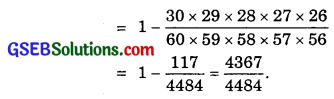Question 2.
4 cards are drawn from a well-shuffled deck of 52 cards. What is the probability of obtaining 3 diamonds and one spade?
Solution:
Out of 52 cards, 4 cards can be drawn in C(52, 4) ways.
There are 13 diamonds cards out of which 3 cards cn be drawn in C(13, 3) ways.
Also, one spade card out of 13 spade cards can be drawn in C(13, 1) ways.
So, favourable number of cases = C(13, 3) × C(13, 1)Question 3.
A die has two faces each with number 1, three faces each with number 2 and one face with number 3. If die is rolled once, determine:
(i) P(2)
(ii) P(1 or 3)
(iii) P(not 3)
Solution:
Total number exhaustive cases = 6.
(i) 3 faces are marked with 2.
Number of favourable cases = 3.
Required probability = P(2) = $$\frac{3}{6}$$ = $$\frac{1}{2}$$.

(ii) 2 faces are marked 1 = P(1) = $$\frac{2}{6}$$.
1 face is marked with 3.
P(3) = $$\frac{1}{6}$$.
∴ P(1 or 3) = $$\frac{2}{6}$$ + $$\frac{1}{6}$$ = $$\frac{3}{6}$$ = $$\frac{1}{2}$$.

(iii) Only 1 face is marked with 3.
5 faces are marked with number which is not 3.
∴ Number of favourable cases = 5.
∴ P(not 3) = $$\frac{5}{6}$$.Question 4.
In a certain lottery, 10000 tickets are sold and ten equal prizes are awarded. What is the probability of not getting a prize, if you buy
(a) 1 ticket?
(b) two tickets?
(c) 10 tickets?
Solution:
Total number of tickets = 10000.
Number of prizes = 10.
∴ Number of tickets which do not carry a prize
= 10000 – 10
= 9990.

(i) Number of ways when 1 ticket without prize is received
= 9990
= 9000C1 = 9000
Total number of tickets = 10000.
∴ Probability of not getting prize with one ticket.
= $$\frac{9990}{10000}$$ = $$\frac{999}{1000}$$.

(ii) Number of ways when 2 tickets without prizes are received.
= 9000C2
2 tickets out of 10000 may be taken out in 10000C2 ways.
∴ Probability of not getting prize with two tickets.
$$\frac{{ }^{9990} \mathrm{C}_{2}}{{ }^{10000} \mathrm{C}_{2}}$$

(iii) 10 tickets out of 9990 may be taken in 9990C10
10 tickets out of 10000 may be taken in 10000C2 ways.
∴ Probability of not getting prize with 10 tickets.
$$\frac{{ }^{9990}{\mathrm{C}}_{10}}{{ }^{10000} \mathrm{C}_{10}}$$Question 5.
Out of 100 students, two sections of 40 and 60 are formed. If you and your friend are among 100 students, what is the probability that
(а) you both enter the same section?
(b) you both enter the different sections?
Solution:
Let there be two sections A and B of 40 and 60 students respectively.
(a) Let both the students enter section A.
∴ 38 students are to be selected out of 98, since two particular students are already in section A.
∴ No. of ways of selecting 38 students out of 98 = 98C35.
No. of exhaustive cases of selecting 40 students out of 100 = 100C40.
∴ Probability that both students enter section A(b) If both the students enter section B.
Then, number of ways of selecting 58 students out of 98 = 98C58
Total number of ways of selecting 60 students out of 100 = 100C60
∴ Probability that the two particular students enter section B∴ Probability that the two particular students enter either Section A or B.
= $$\frac{26}{125}$$ – $$\frac{59}{100}$$ – $$\frac{85}{100}$$ – $$\frac{17}{33}$$
The probability that they enter different sections
= 1 – $$\frac{17}{33}$$ = $$\frac{16}{33}$$.

Question 6.
Three letters are dictated to three persons and an envelope is addressed to each of them, the letters are inserted into the envelopes at random so that each envelope contains exactly one letter. Find the probability that at least one letter is in its proper envelope.
Solution:
Let the envelope be denoted by A, B and C and the corresponding letters by a, b and c.
1 letter in correct envelope and 2 in wrong envelope may be put as
(Aa, Bc, Cb), (Ac, Bb, Ca), (Ab, Ba, Cc).
Two letters or consequently all the letters are in correct envelope may be put up in one way, i.e., (Aa, Bb, Cc).
∴ Number of exhaustive cases = 3! = 6 ways.
Number of favourable cases = 4.
Probability that at least one letter is in its proper envelope
= $$\frac{4}{6}$$ = $$\frac{2}{3}$$.Question 7.
A and B are two events such that P(A) = 0.54, P(B) = 0.69, and P(A ∩ B) = 0.35. Find:
(i) P(A ∪ B)
(ii) P(A’ ∩ B’)
(iii) P(A ∩ B’)
(iv) P(B ∩ A’)
Solution:
(i) P(A ∪ B) = P(A) + P(B) – P(A ∩ B)
= 0.54 + 0.69 – 0.35
= 1.23 – 0.35
= 0.88.

(ii) A’ ∩ B’ = (A ∪ B)’ [By De Morgan’s law]
∴ P(A’ ∩ B ’) = P(A ∪ B)’ = 1 – P(A ∪ B)
= 1 – 0.88
= 0.12.

(iii) A ∩ B’ = A – A ∩ B
∴ P(A ∩ B’) = P(A) – P( A ∩ B)
= 0.54 – 0.35
= 0.19.

(iv) P(B ∩ A’) = P(B) – P(A ∩ B)
= 0.69 – 0.35 = 0.34.

Question 8.
From the employees of a company, 5 persons are selected to represented them in the managing committee of the company. particulars of five persons around follows: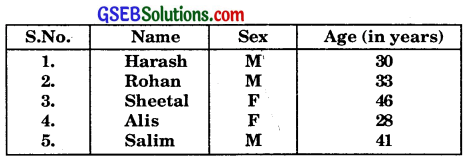A person is selected at random from this group to act as a spokes person. What is the probability that the spokes person will be either male or over 35 years?
Solution:
Let A denotes the event of selection of male and B denotes the event of selection of person over 35 years.
∴ P(A) = $$\frac{C(3, 1)}{C(5, 1)}$$ = $$\frac{3}{5}$$.
P(B) = $$\frac{C(3, 1)}{C(5, 1)}$$ = $$\frac{2}{5}$$
[∵ There are 2 persons over 35 years]
and P(A ∩ B) = $$\frac{1}{5}$$.
∴ P(either male or over 35 years)
= P(A ∪ B)
= P(A) + P(B) – P(A ∩ B)
= $$\frac{3}{5}$$ + $$\frac{2}{5}$$ – $$\frac{1}{5}$$ = $$\frac{4}{5}$$.Question 9.
If 4-digit numbers greater than 5000 are randomly formed from the digits 0,1, 3, 5 and 7, what is the probability of forming a number divisible by 5, when
(i) the digits may be repeated?
(ii) the repetition of digits is not allowed?
Solution:
Let 4 places in a 4-digit number be named as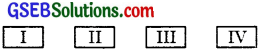(i) When digits are repeated:
If place I is filled up with 5, the places II, III, IV may be filled up in 5 × 5 × 5 = 125 ways.
Similarly, when place I is filled up with 7, again places II, III, IV may be filled up in 5 × 5 × 5 = 125 ways.
When 4-digit number is greater than 5000, the number of exhaustive cases = 125 + 125 = 250.
A number is divisible by 5 in four cases: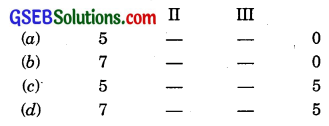In each case, II and III places may be filled up in 5 × 5 = 25 cases.
∴ Number of numbers which are divisible by 5 = 4 × 25 = 100.
∴ When repetition of digits is allowed,
probability that number is greater than 5000 and divisible by 5 = $$\frac{100}{250}$$ = $$\frac{2}{5}$$

(ii) When digits are not repeated :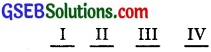Let place I be filled up with 5.
Place II, III, IV may be filled up in 4 × 3 × 2 = 24 ways.
Similarly, when 7 is placed at I, again II, III, IV places may be filled up in 4 × 3 × 2 = 24 ways.Total number cases, where 4-digit number is greater than 5000 = 24 + 24 = 48.
Now, a number is divisible by 5 is obtained when 0 or 5 is placed at IV asIn each case, II and III places can be filled up in 3 × 2 = 6 ways
When digits are not repeated, the number of numbers divisible by 5 are 6 + 6 + 6 = 18.
∴ Probability that 4-digits number greater than 5000 and divisible by 5 = $$\frac{18}{48}$$ = $$\frac{3}{8}$$.Question 10.
The number lock of a suit case has four wheels, each labelled with 10 digits, i.e., from 0 to 9.
The lock opens with a sequence of four digits with no repeats. What is the probability of a person getting the right sequence to open the suitcase.
Solution:
When digits are not repeated, the first place may have one of the 10 digits, the second 9, third 8, fourth 7.
∴ No. of 4 digit numbers (including zero of thousands places) are
10 × 9 × 8 × 7 = 5040.
∴ Now the lock may be opened only in 1 way.
∴ Probability of opening the lock = $$\frac{1}{5040}$$.